# Instantaneous Velocity Formula

Instantaneous Velocity Formula

Velocity is a measure of how quickly an object moves from one position to another. If an object is accelerating or decelerating, the velocity of the object changes with time. The instantaneous velocity of an object is the velocity at a certain instant of time. Velocity is the change in position divided by the change in time, and the instantaneous velocity is the limit of velocity as the change in time approaches zero. This is equivalent to the derivative of position with respect to time. Instantaneous velocity is a vector, and so it has a magnitude (a value) and a direction. The unit for instantaneous velocity is meters per second (m/s).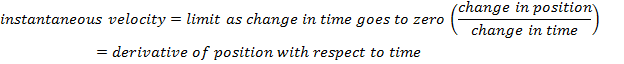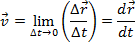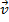= instantaneous velocity (m/s)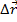= vector change in position (m)

Δt = change in time (s)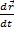= derivative of vector position with respect to time (m/s)

Instantaneous Velocity Formula Questions:

1) A cat that is walking toward a house along the top of a fence is moving at a varying velocity. The cat's position on the fence is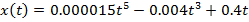. Position x is in meters, and time t is in seconds. What is the cat's instantaneous velocity at time t = 10.0 s?

Answer: The cat's velocity can be found using the formula:The cat's position has only one component, since it is moving in a straight line along the fence. The positive x direction is chosen to be toward the house. The magnitude of the velocity in the x direction is: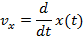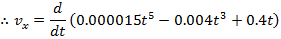This derivative can be solved using the scalar multiple rule and the power rule for derivatives: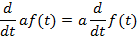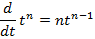The magnitude of the instantaneous velocity is: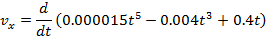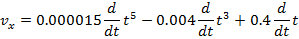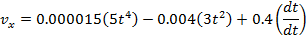The magnitude of the cat's instantaneous velocity at t = 10.0 s is: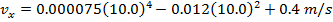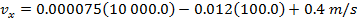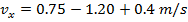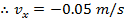The cat's instantaneous velocity at t=10.0 s is 0.05 m/s in the -x direction (away from the house).

2) A child kicks a ball horizontally, off the edge of a cliff. The horizontal position of the ball is given by the function x(t) = bt, where b = 6.0 m/s. The vertical position of the ball is given by the function y(t) = ct2, where c = -4.90 m/s2. At t = 4.0 s, what are the horizontal and vertical components of the instantaneous velocity?

Answer: The components of the instantaneous velocity can be found using the formula:If the horizontal direction of the ball is defined as the positive x direction, and vertically upward is defined as the positive y direction, then the magnitudes of the x and y components of the instantaneous velocity are: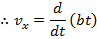and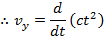These can be solved using the scalar multiple rule and the power rule for derivatives:The horizontal instantaneous velocity is: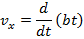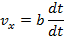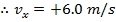The horizontal velocity of the ball is a constant value of 6.0 m/s in the +x direction.

The vertical instantaneous velocity is: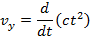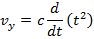vy = c(2t)

vy = 2ct

At t = 4.0 s, the vertical instantaneous velocity is:

vy = 2ct

vy = 2(-4.90 m/s2)(4.0 s)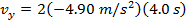The vertical instantaneous velocity at t = 4.0 s is 39.2 m/s in the -y direction.